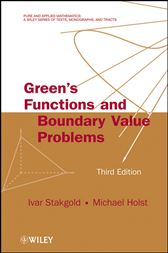Green's Functions and Boundary Value Problems (3rd ed.)

Series: Pure and Applied Mathematics: A Wiley Series of Texts, Monographs and Tracts (No. 99)CA\$197.25
CA\$177.53
ISBNs
• 0470906529
• 9780470609705
• 9780470906521
Praise for the Second Edition

"This book is an excellent introduction to the wide field of boundary value problems."—Journal of Engineering Mathematics

"No doubt this textbook will be useful for both students and research workers."—Mathematical Reviews

A new edition of the highly-acclaimed guide to boundary value problems, now featuring modern computational methods and approximation theory

Green's Functions and Boundary Value Problems, Third Edition continues the tradition of the two prior editions by providing mathematical techniques for the use of differential and integral equations to tackle important problems in applied mathematics, the physical sciences, and engineering. This new edition presents mathematical concepts and quantitative tools that are essential for effective use of modern computational methods that play a key role in the practical solution of boundary value problems. With a careful blend of theory and applications, the authors successfully bridge the gap between real analysis, functional analysis, nonlinear analysis, nonlinear partial differential equations, integral equations, approximation theory, and numerical analysis to provide a comprehensive foundation for understanding and analyzing core mathematical and computational modeling problems.

Thoroughly updated and revised to reflect recent developments, the book includes an extensive new chapter on the modern tools of computational mathematics for boundary value problems. The Third Edition features numerous new topics, including:

• Nonlinear analysis tools for Banach spaces

• Finite element and related discretizations

• Best and near-best approximation in Banach spaces

• Iterative methods for discretized equations

• Overview of Sobolev and Besov space linear

• Methods for nonlinear equations

• Applications to nonlinear elliptic equations

In addition, various topics have been substantially expanded, and new material on weak derivatives and Sobolev spaces, the Hahn-Banach theorem, reflexive Banach spaces, the Banach Schauder and Banach-Steinhaus theorems, and the Lax-Milgram theorem has been incorporated into the book. New and revised exercises found throughout allow readers to develop their own problem-solving skills, and the updated bibliographies in each chapter provide an extensive resource for new and emerging research and applications.

With its careful balance of mathematics and meaningful applications, Green's Functions and Boundary Value Problems, Third Edition is an excellent book for courses on applied analysis and boundary value problems in partial differential equations at the graduate level. It is also a valuable reference for mathematicians, physicists, engineers, and scientists who use applied mathematics in their everyday work.

ISBNs
• 0470906529
• 9780470609705
• 9780470906521